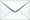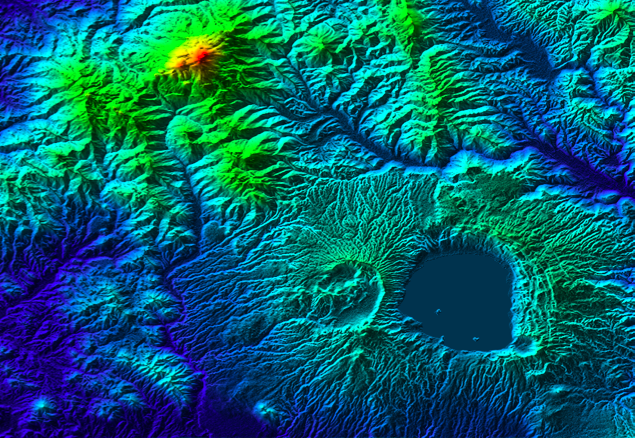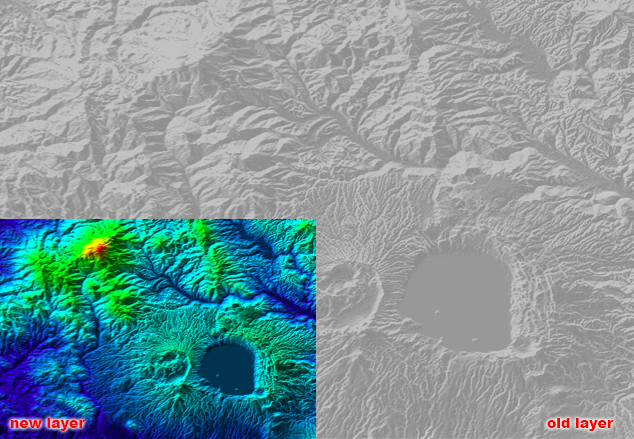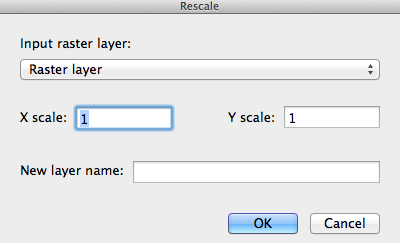Product Search

Dear customers:

take action as soon as possible.tyevolution@yahoo.com

# M: Tool Box__Raster__Raster transformation__Rescale

Views:22times

Summarize:

Resizes a raster by the specified x and y scale factors.

Usage:

•  The input layers must be raster layers.  The names of all input layers must be unique.

•  The output size is multiplied by the scale factor for both the x and y directions. The number of columns and rows stays the same in this process, but the cell size is multiplied by the scale factor.

•  The scale factor must be positive.

•  A scale factor greater than one means the image will be rescaled to a larger dimension, resulting in a larger

extent because of a larger cell size.

•  A scale factor less than one means the image will be rescaled to a smaller dimension, resulting in a smaller

extent because of a smaller cell size.

Diagram:

The input layerThe output layerDialog: